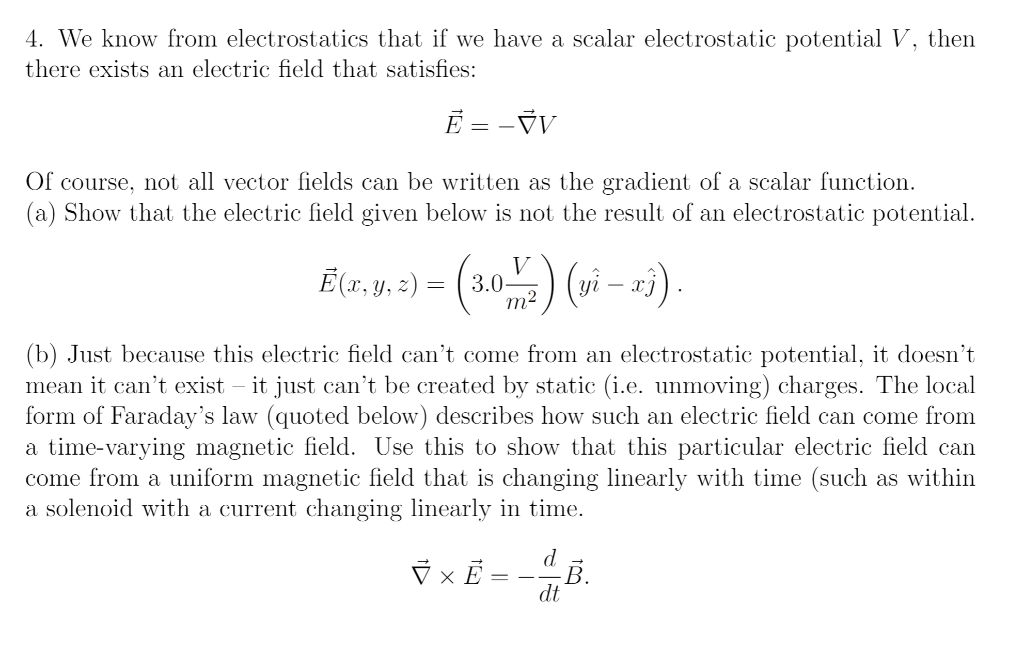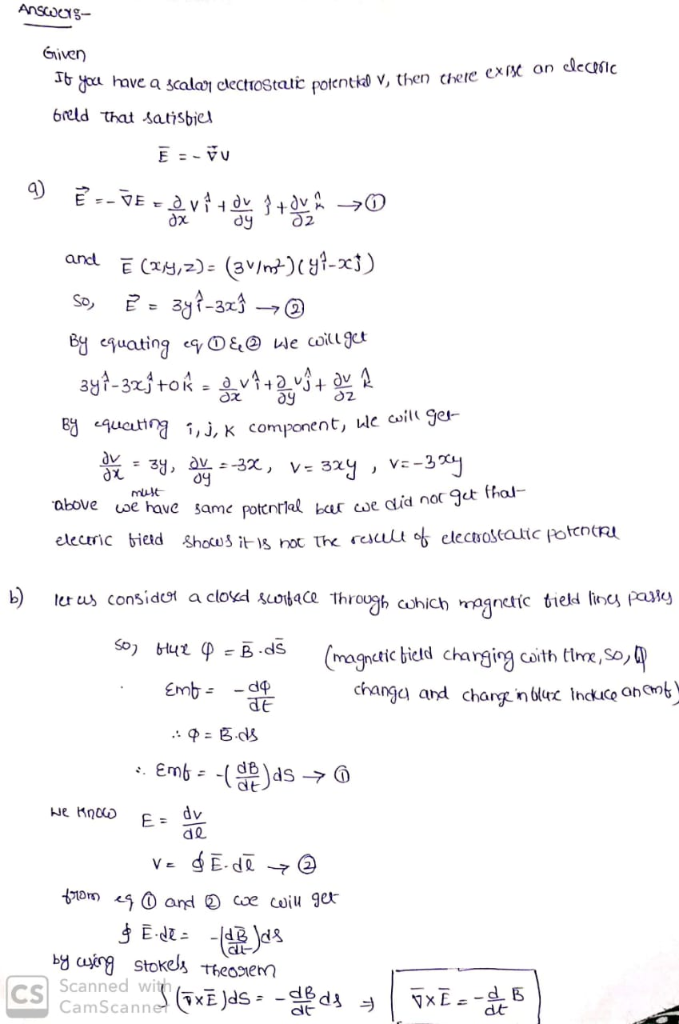# 4. We know from electrostatics that if we have a scalar electrostatic potential V, then there exists an electric field...4. We know from electrostatics that if we have a scalar electrostatic potential V, then there exists an electric field that satisfies: Of course, not all vector fields can be written as the gradient of a scalar function. (a) Show that the electric field given below is not the result of an electrostatic potential (b) Just because this electric field can't come from an electrostatic potential, it doesn't mean it can't exist - it just can't be created by static (i.e. unmoving) charges. The local form of Faraday's law (quoted below) describes how such an electric field can come from a time-varying magnetic field. Use this to show that this particular electric field can come from a uniform magnetic field that is changing linearly with time (such as within a solenoid with a current changing linearly in time. dt##### Add Answer of: 4. We know from electrostatics that if we have a scalar electrostatic potential V, then there exists an electric field...
More Homework Help Questions Additional questions in this topic.